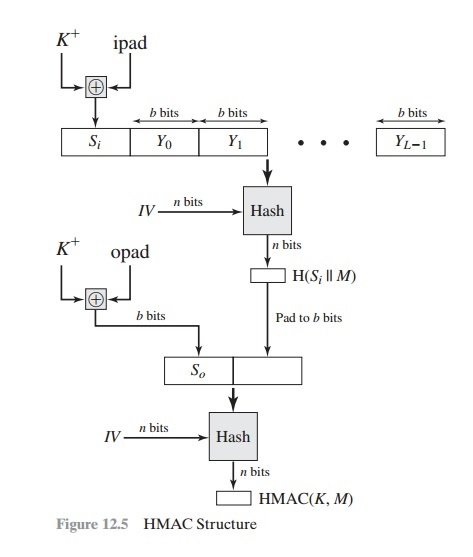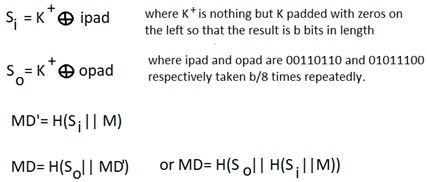# MAC-based on Hash Function (HMAC) in Cryptography

Cryptography | MAC-based on Hash Function (HMAC): In this tutorial, we will briefly study the basis of HMAC and examples aim to capture. We will cover types of messages in HMAC. By Monika Sharma Last updated : May 25, 2023

## What is MAC-based on Hash Function (HMAC) in Cryptography?

This Hashed or Hash-based Message Authentication Code in cryptography. Thus, developing a MAC derived from cryptographic hash functions in this. Mainly, HMAC is a great resistance towards cryptanalysis attacks as it uses the hashing concept twice in cryptography.

Thus, HMAC consists of twin benefits of Hashing and MAC and thus is more secure than any other authentication code in cryptography. Thus, RFC 2104 has issued HMAC, and HMAC has been made compulsory to implement in IP security in MAC.

Then, the FIPS 198 NIST standard has also issued HMAC in cryptography.

## HMAC Algorithm

Hence, the working of HMAC starts with taking a message M containing blocks of length b bits of the message. Thus, an input signature is padded to the left of the message and the whole is given as input to a hash function which gives us a temporary message-digest MD' of the message of plaintext or original text.

Thus, MD' again is appended to an output signature, and the whole is applied a hash function again, the result is our final message digest MD in cryptography.

## Block Diagram of SHA-1• Here, H = Hashing function,
• M is plaintext or original text message
• Here, Si and So are input and output signatures respectively,
• Yi is the ith block in the original text or plaintext message M, where i ranges from [1, L)
• L = the count of blocks in M
• K is the secret key used for hashing value
• IV is an initial vector as some constant
• Mainly, the generation of input signature and output signature Si and So respectively.We can describe the algorithm as follows,

Thus, append zeros to the left end of K to create a b-bit string K+ (e.g., if K is of length 160 bits and b = 512, then K will be appended with 44 zeroes) in this.

1. Then, XOR (bitwise exclusive-OR) K+ with iPad to produce the b-bit block Si.
2. Now, append M to Si.
3. Then, apply H to the stream generated in step 3.
4. Then, XOR K+ with opad to produce the b-bit block So.
5. And append the hash result from step 4 to So.
6. And apply H to the stream generated in step 6 and output the result.

Therefore, the XOR with opad results in flipping one-half of the bits of K, using a different set of bits of the message. Since, bypassing Si and So through the compression function of the hash algorithm, we have pseudo-randomly generated two keys from K in cryptography.

Thus, HMAC should execute at approximately the same time as the embedded hash function for long messages of plaintext or original text. Then, HMAC adds three executions of the hash compression function (for Si, So, and the block produced from the inner hash) in cryptography.

## Security of HMAC

Mainly, the secure security of the hash function depends in some way on the cryptographic strength of the underlying hash function in this algorithm. Then, the appeal of HMAC is that its designers have been able to hash function and the strength of HMAC in this algorithm.

As we see that the secure security of a MAC function is generally expressed in terms of the probability of time spent by the forger and a given number of messages–tag pairs created with the same key in cryptography. Mainly, it is solved in that for a given level of effort on messages generated by a legitimate user and seen by the attacker, the probability of a successful attack on HMAC is equivalent to one of the following attacks on the embedded hash function in cryptography as follow,

1. Thus, the attacker or hacker is able to compute the output of the compression function even with an IV that is random, secret, and unknown to the attacker in this.
2. Thus, the attacker finds collisions in the hash function even when the IV is random and secret in cryptography.

Hence, a normal hash function HMAC adds a compression instance to the processing in this. And, this structural implementation holds efficiency for shorter MAC values in cryptography.

Reference: HMAC